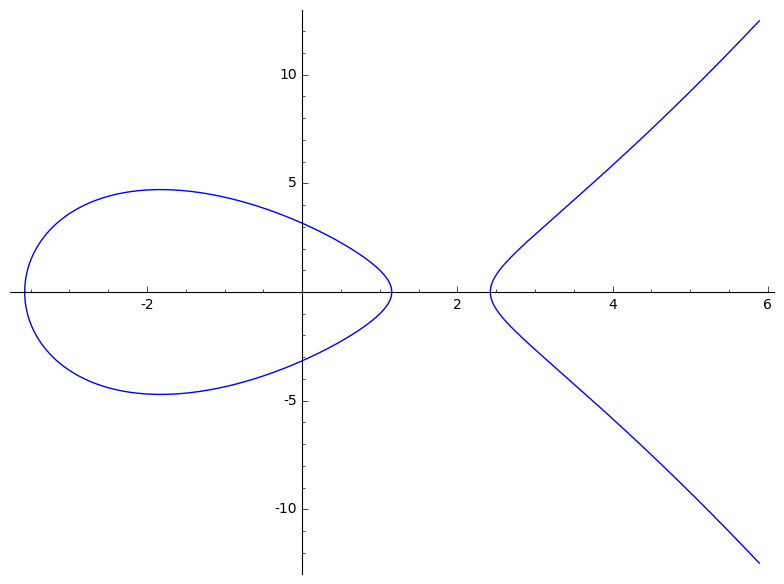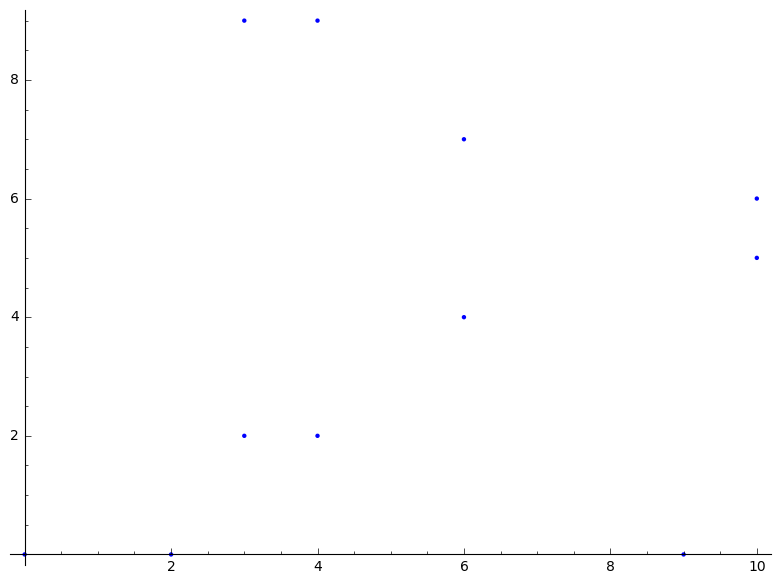# Using elliptic curves and isogenies in SageMath¶

This is a short tutorial to get started with elliptic curves in SageMath. For a complete reference, see the official documentation:

## Finite fields¶

We create finite fields by passing their cardinality

In :
Fp = GF(11)

In :
Fp

Out:
Finite Field of size 11
In :
Fq = GF(11^2)
Fq

Out:
Finite Field in z2 of size 11^2

For extension fields, the generator is obtained with the .gen() function.

In :
z = Fq.gen()
z

Out:
z2
In :
z^120

Out:
1

Same thing in one go

In :
K.<t> = GF(next_prime(2^128)^2)
K

Out:
Finite Field in t of size 340282366920938463463374607431768211507^2

## Elliptic curves¶

Curves over $ℚ$

In :
E = EllipticCurve([-10,10])
E

Out:
Elliptic Curve defined by y^2 = x^3 - 10*x + 10 over Rational Field
In :
E.plot()

Out:Cuvers over other fields

In :
F = EllipticCurve(GF(11), [1, 0])
F

Out:
Elliptic Curve defined by y^2 = x^3 + x over Finite Field of size 11
In :
F.order()

Out:
12
In :
F.cardinality()

Out:
12
In :
F.points()

Out:
[(0 : 0 : 1), (0 : 1 : 0), (5 : 3 : 1), (5 : 8 : 1), (7 : 3 : 1), (7 : 8 : 1), (8 : 5 : 1), (8 : 6 : 1), (9 : 1 : 1), (9 : 10 : 1), (10 : 3 : 1), (10 : 8 : 1)]
In :
P = F.random_point()
P

Out:
(5 : 3 : 1)
In :
P.order()

Out:
3

Isomorphisms

In :
F.automorphisms()

Out:
[Generic endomorphism of Abelian group of points on Elliptic Curve defined by y^2 = x^3 + x over Finite Field of size 11
Via:  (u,r,s,t) = (1, 0, 0, 0),
Generic endomorphism of Abelian group of points on Elliptic Curve defined by y^2 = x^3 + x over Finite Field of size 11
Via:  (u,r,s,t) = (10, 0, 0, 0)]
In :
aut = F.change_ring(GF(11^2)).automorphisms()
aut

Out:
[Generic endomorphism of Abelian group of points on Elliptic Curve defined by y^2 = x^3 + x over Finite Field in z2 of size 11^2
Via:  (u,r,s,t) = (1, 0, 0, 0),
Generic endomorphism of Abelian group of points on Elliptic Curve defined by y^2 = x^3 + x over Finite Field in z2 of size 11^2
Via:  (u,r,s,t) = (10, 0, 0, 0),
Generic endomorphism of Abelian group of points on Elliptic Curve defined by y^2 = x^3 + x over Finite Field in z2 of size 11^2
Via:  (u,r,s,t) = (4*z2 + 3, 0, 0, 0),
Generic endomorphism of Abelian group of points on Elliptic Curve defined by y^2 = x^3 + x over Finite Field in z2 of size 11^2
Via:  (u,r,s,t) = (7*z2 + 8, 0, 0, 0)]
In :
aut, aut^2

Out:
(Generic endomorphism of Abelian group of points on Elliptic Curve defined by y^2 = x^3 + x over Finite Field in z2 of size 11^2
Via:  (u,r,s,t) = (7*z2 + 8, 0, 0, 0),
Generic endomorphism of Abelian group of points on Elliptic Curve defined by y^2 = x^3 + x over Finite Field in z2 of size 11^2
Via:  (u,r,s,t) = (10, 0, 0, 0))
In :
G = EllipticCurve(GF(11), [3, 0])
F.is_isomorphic(G)

Out:
True
In :
u = F.isomorphism_to(G)
u

Out:
Generic morphism:
From: Abelian group of points on Elliptic Curve defined by y^2 = x^3 + x over Finite Field of size 11
To:   Abelian group of points on Elliptic Curve defined by y^2 = x^3 + 3*x over Finite Field of size 11
Via:  (u,r,s,t) = (8, 0, 0, 0)
In :
P, u(P)

Out:
((5 : 3 : 1), (3 : 6 : 1))

Group structure

In :
F.abelian_group()

Out:
Additive abelian group isomorphic to Z/12 embedded in Abelian group of points on Elliptic Curve defined by y^2 = x^3 + x over Finite Field of size 11
In :
g = F.gens()
g

Out:
(8 : 5 : 1)
In :
g.order()

Out:
12

Construct an isogeny with given kernel

In :
origin = 6*g
origin

Out:
(0 : 0 : 1)
In :
F.point([0,0])

Out:
(0 : 0 : 1)
In :
I = F.isogeny(origin)
I

Out:
Isogeny of degree 2 from Elliptic Curve defined by y^2 = x^3 + x over Finite Field of size 11 to Elliptic Curve defined by y^2 = x^3 + 7*x over Finite Field of size 11
In :
I.rational_maps()

Out:
((x^2 + 1)/x, (x^2*y - y)/x^2)
In :
FF = I.codomain()

In :
FF

Out:
Elliptic Curve defined by y^2 = x^3 + 7*x over Finite Field of size 11
In :
FF.abelian_group()

Out:
Additive abelian group isomorphic to Z/6 + Z/2 embedded in Abelian group of points on Elliptic Curve defined by y^2 = x^3 + 7*x over Finite Field of size 11
In :
FF.plot()

Out:The same example, over the rationals

In :
E = EllipticCurve([1,0])

In :
P = E.lift_x(0)
P

Out:
(0 : 0 : 1)
In :
P.order()

Out:
2
In :
J = E.isogeny(P)
EE = J.codomain()
EE

Out:
Elliptic Curve defined by y^2 = x^3 - 4*x over Rational Field

In (very) limited cases, Sage can compute the isogeny given the image curve and the degree

In :
JJ = E.isogeny(None, codomain=EE, degree=2)

In :
J == JJ

Out:
True

## Orders¶

The functionality is a bit limited, but still useful

In :
E = EllipticCurve([1,0])
E

Out:
Elliptic Curve defined by y^2 = x^3 + x over Rational Field
In :
E.has_cm()

Out:
True
In :
E.cm_discriminant()

Out:
-4

Over finite fields

In :
E = EllipticCurve(GF(101), [1,2])
E

Out:
Elliptic Curve defined by y^2 = x^3 + x + 2 over Finite Field of size 101
In :
E.j_invariant()

Out:
4
In :
chi = E.frobenius_polynomial()
chi

Out:
x^2 - 2*x + 101
In :
chi.discriminant()

Out:
-400
In :
E.trace_of_frobenius()

Out:
2
In :
O = E.frobenius_order()
O

Out:
Order in Number Field in phi with defining polynomial x^2 - 2*x + 101
In :
O.is_maximal()

Out:
False
In :
O.discriminant()

Out:
-400
In :
K = O.number_field()
K

Out:
Number Field in phi with defining polynomial x^2 - 2*x + 101
In :
OK = K.maximal_order()
OK

Out:
Gaussian Integers in Number Field in phi with defining polynomial x^2 - 2*x + 101
In :
OK.discriminant()

Out:
-4
In :
K.class_number()

Out:
1
In :
K.class_group()

Out:
Class group of order 1 of Number Field in phi with defining polynomial x^2 - 2*x + 101
In :
O.class_number()

Out:
4
In :
H = hilbert_class_polynomial(-400)
H

Out:
x^4 - 1938773508354872717845384224*x^3 + 12869286863161864184636279443710336*x^2 - 19075061455767889406477974994607212544*x + 87448873738295790450948276123544550117376
In :
H.is_irreducible()

Out:
True
In :
H.change_ring(GF(101)).factor()

Out:
(x + 7) * (x + 24) * (x + 64) * (x + 97)

## Exercises¶

### Give the list of all elliptic curves with complex multiplication over $ℚ$¶

In [ ]:



### Give the list of all supsersingular elliptic curves over $𝔽_{101^2}$¶

In [ ]:



### Find a prime $p$ and an elliptic curve $E/𝔽_p$ such that $\#E(𝔽_p) = 101$.¶

Hint: try to find a solution to $p+1-t = 101$, then compute the discriminant of the Frobenius and use complex multiplication theory.

In [ ]:



### Use the previously found curve to construct an isogeny of degree $101^{10}$¶

In [ ]:



### Find a prime $p$ and an elliptic curve $E/𝔽_p$ such that $\#E(𝔽_p) = 2^{127} - 1$¶

Warning: this is obviously a difficult exercise, and requires knowledge not contained in the course (for example, of Cornacchia's algorithm)

In [ ]: